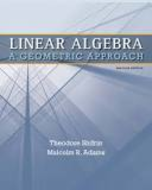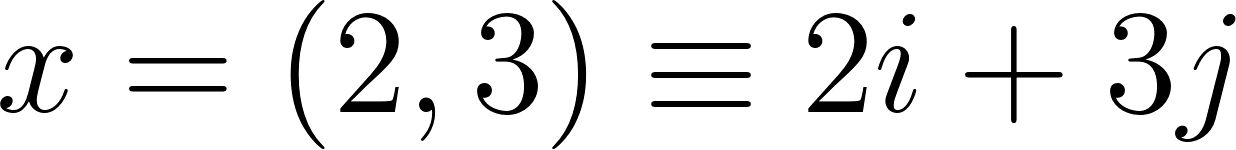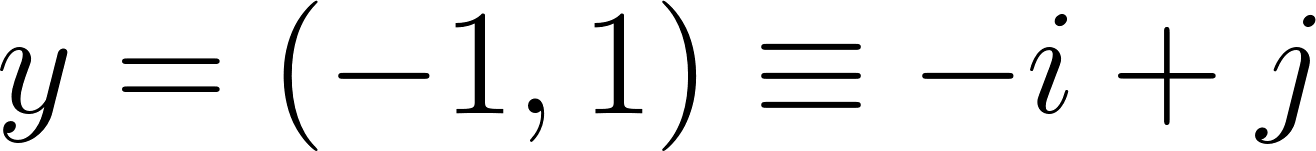### Solution Found!# Given x = (2, 3) and y = (1, 1), calculate the following algebraically and sketch a

Chapter 1, Problem 1

(choose chapter or problem)

Get Unlimited Answers! Check out our subscriptions
QUESTION:

1. Given x = (2, 3) and $$y=(-1,1)$$, calculate the following algebraically and sketch a picture to show the geometric interpretation.

a. x + y

b. $$\mathbf{x}-\mathbf{y}$$

c. x + 2y

d. $$\frac{1}{2} \mathbf{x}+\frac{1}{2} \mathbf{y}$$

e. $$y-x$$

f. $$2 \mathbf{x}-\mathbf{y}$$

g. $$\|\mathbf{x}\|$$

h. $$\frac{\mathbf{x}}{\|\mathbf{x}\|}$$

QUESTION:

1. Given x = (2, 3) and $$y=(-1,1)$$, calculate the following algebraically and sketch a picture to show the geometric interpretation.

a. x + y

b. $$\mathbf{x}-\mathbf{y}$$

c. x + 2y

d. $$\frac{1}{2} \mathbf{x}+\frac{1}{2} \mathbf{y}$$

e. $$y-x$$

f. $$2 \mathbf{x}-\mathbf{y}$$

g. $$\|\mathbf{x}\|$$

h. $$\frac{\mathbf{x}}{\|\mathbf{x}\|}$$

Step 1 of  9